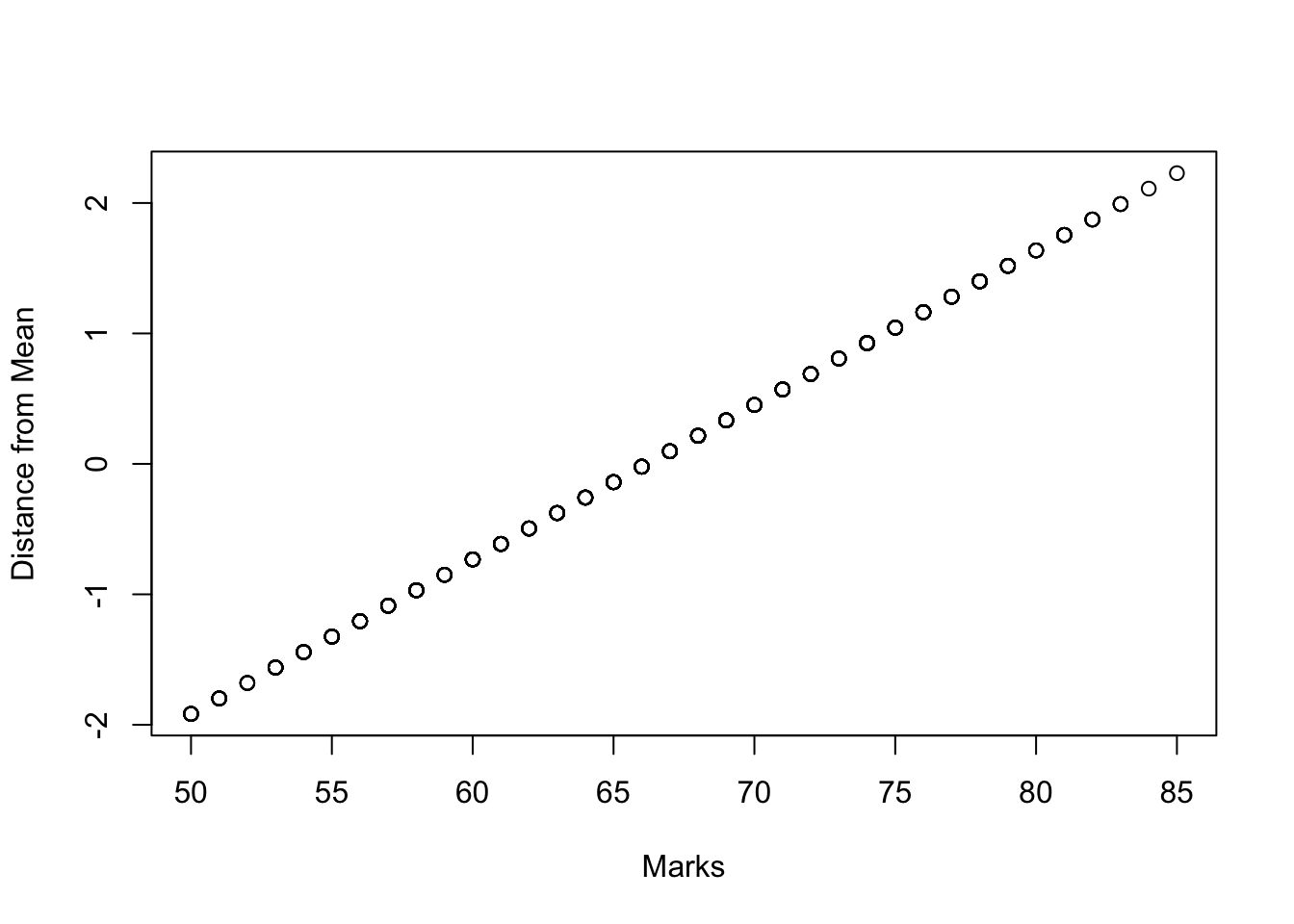In the last session we have just shown how to develop a stronger degree of confidence in the height of the students. But when probablities are involved there is always a big chance that we get it wrong - even with a larger population. Canning (2014) explains that whatever our level of confidence we can make still make two possible errors. The first one rejects the null hypothesis when it is actully true. This kind of error if called a false positive and assumes, for instance, that you do not pass a difficult module when you actually have passed it. In statistics, this is called a Type I error. The second error we can make is the false negative where we accept the null hypothesis when it is actually false. In statistics this is called a Type II error.

To avoid these errors, all statistical reasoning will deliver a set of summary values that allow you to assess the risk that you got it wrong. In R, these are often produced at the same time as the results of the inference. The most commonly cited value to verify the results of statistical inference is the p-value. The p-value approach involves determining “likely” or “unlikely” by determining the probability — assuming the null hypothesis were true — of observing a more extreme test statistic in the direction of the alternative hypothesis than the one observed. If the p-value is small, then it is unlikely. If the p-value is large then it is likely. Let’s go through an example next to make this clearer and in particular what a large p-value is.

Let us assume, for instance, that we want to find out whether it is common to get 80 in the module. This means our null hypothesis is that it is common to get 80 in this module. To get the p-value, we first fit the existing distribution onto our old friend the normal distribution by shifting the centre of the curve to the mean and then use the standard deviation to calculate the distance from this centre. https://statistics.laerd.com/statistical-guides/standard-score.php explains this process very well.

The p-value generally describes the point on the normal distribution’s curve, where we reach the very unlikely results. We can calculate the p-value of 80 using R’s pnorm which takes the value, the mean and the standard deviation (https://www.r-bloggers.com/normal-distribution-functions/). We need to subtract it from one to get the part of the normal curve, which describes the highly unlikely results. Run 1 - pnorm(80, mean(student_survey\(Marks), sd(student_survey\)Marks)) to calculate this right-hand p-value.

``1 - pnorm(80, mean(student_survey\$Marks), sd(student_survey\$Marks))``
``##  0.05086819``

You will get a back a value of around 0.05. Often, statisticians will define the significance level to be at 5% (or 0.05). If the p-value is less than 5%, then the null hypothesis is rejected and it is not common to score 80 marks in this module. As we reach a value of around 5% (0.05), we can say that that the null hypothesis can be rejected and it is not common to reach 80. It is too far away from the mean to count to the common cases. An excellent further explanation of the p-value can be found at http://www.dummies.com/education/math/statistics/what-a-p-value-tells-you-about-statistical-data/.

The p-value is thus using mean and standard deviation to relate the empirical obserservations and their distribution to the normal distribuion. If the p-value is very small, it will be very unlikely that there is only a random relationship. This almost concludes our discussion in this section. But before we move on to the next topic, we would like to introduce one final concept related to the normal distribution. The z-score helps with standardising a distribution.

A popular way of standardising the values of a distribution is the z-score, which fits the current distribution to its mean and distances from the mean, given by multiples of standard deviations. Run plot(student_survey\(Marks, scale(student_survey\)Marks), xlab = “Marks”, ylab = “Distance from Mean”). You will see what I mean with regard to the marks. The z-score is also called standard score because it represents any value as a standardised distance from the mean. z-scores and p-values often work in conjunction.

``plot(student_survey\$Marks, scale(student_survey\$Marks), xlab = "Marks", ylab = "Distance from Mean")``This concludes our discussion of statistical inference for a single value such as the students’ marks or heights. Next we will continue with statistical inference of the relationship of two or more variables and their groups.

We move on now to investigating how two variables relate to each other using inferential statistics. First we investigate the correlation of two numerical variables (https://en.wikipedia.org/wiki/Correlation_and_dependence) and the dependence it shows. In particular, we will analyse the relationship between the hours spent on the class to the marks. Check out Chapter 13 of Canning (2014) for an overview of understanding variable relationships with statistics. Further statistical test we introduce in this part are z-test, t-test, chi-square and Analysis of Variance (ANOVA). All of these are designed to work out relationships in different context such as for discrete or continuous variables or for dependent or independent variables. A statistical test always helps us to identify differences between two or more groups of data. In our case, we could, for instance, evaluate the module performance in terms of differences in the gender of the students.

Our survey data also contains information about how many hours a week the students have spent on the module. Now, we can investigate whether those students that spent more time on the class also got better marks. Plot the hours in relation to the marks with plot(student_survey\(Preparation_Hours, student_survey\)Marks, col = “red”, xlab = “Hours”, ylab = “Mark”, main = “Student Time spent for a Mark”).

``plot(student_survey\$Preparation_Hours, student_survey\$Marks, col = "red", xlab = "Hours", ylab = "Mark", main = "Student Time spent for a Mark")``